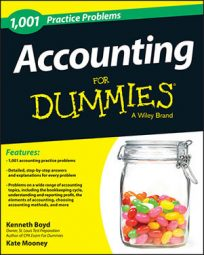##### AccountingThe following are some of the most frequently used accounting formulas. This list is not comprehensive, but it should cover the items you’ll use most often as you practice solving various accounting problems.

## Balance sheet formula

Assets – liabilities = equity (or assets = liabilities + equity)

This basic formula must stay in balance to generate an accurate balance sheet. This means that all accounting transactions must keep the formula in balance. If not, the accountant has made an error.

## Retained earnings formula

Beginning balance + net income – net losses – dividends = ending balance

## Income statement formula

Revenue (sales) – expenses = profit (or net income)

Keep in mind that revenue and sales may be used interchangeably. Profit and net income may also be used interchangeably. The income statement is also referred to as a profit and loss statement.

## Gross margin

Sales – cost of sales

Gross margin is not a company’s net income or profit. Other expenses, such as selling, general, and administrative (SG and A) expenses, are subtracted to arrive at net income.

## Operating income (earnings)

Gross profit – selling, general, and administrative (SG and A) expenses

## Statement of cash flows formula

Beginning cash balance + cash flow sources (uses) from operations + cash flow sources (uses) from financing + cash flow sources (uses) from investing = ending cash balance

This formula adds cash sources and subtracts cash uses.

## Inventory formula

Beginning inventory + purchases – cost of sales = ending inventory (or beginning inventory + purchases – ending inventory = cost of sales)

## Net sales formula

Gross sales – sales discounts – sales returns and allowances

## Book value of fixed (depreciable) assets

Original cost – accumulated depreciation

## Straight line depreciation

(Original cost – salvage value) / number of years in useful life

Salvage value is the dollar amount that the owner can receive for selling the asset at the end of its useful life.